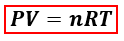### Learn how to solve problems like this one with our expert tutor.

🤓 Based on our data, we think this question is relevant for Professor Nair's class at TAMU.

# Solution: The combustion of octane, C8H18, proceeds according to the reaction2C8H18(l) + 25O2(g) → 16CO2(g) + 18H2O(l) If 305 mol of octane combusts, what volume of carbon dioxide is produced at 35.0°C and 0.995 atm?

###### Problem

The combustion of octane, C8H18, proceeds according to the reaction

2C8H18(l) + 25O2(g) → 16CO2(g) + 18H2O(l)

If 305 mol of octane combusts, what volume of carbon dioxide is produced at 35.0°C and 0.995 atm?

###### Solution

We are asked to calculate the volume of carbon dioxide (CO2) produced from the combustion of octane (C8H18). We can calculate the volume of the gas using the ideal gas equation:P = pressure, atm
V = volume, L
n = moles, mol
R = gas constant = 0.08206 (L·atm)/(mol·K)
T = temperature, K

In the ideal gas equation, the amount of CO2 in moles is needed but the moles of C8H18 is given instead. We will have to calculate the moles of CO2 produced from CH4 first using the balanced reaction equation and mole to mole comparison.View Complete Written Solution